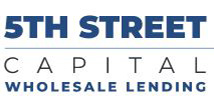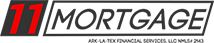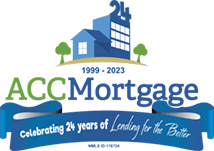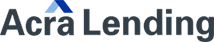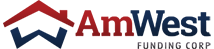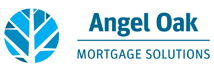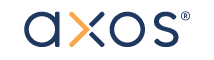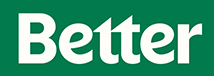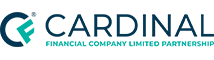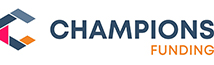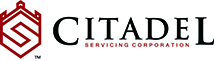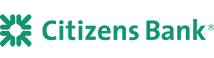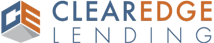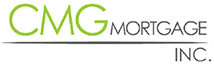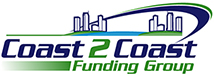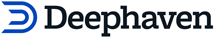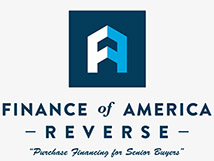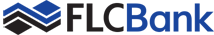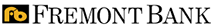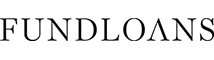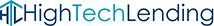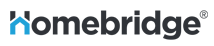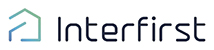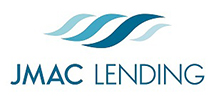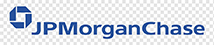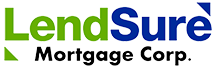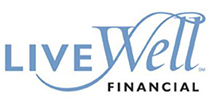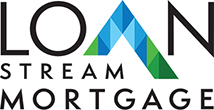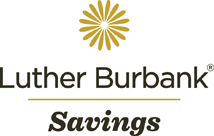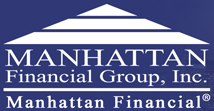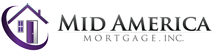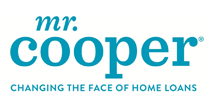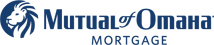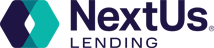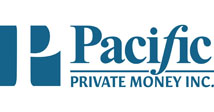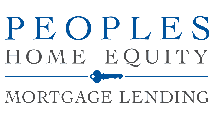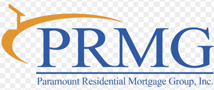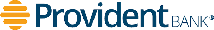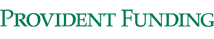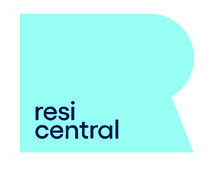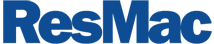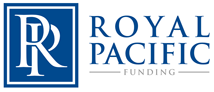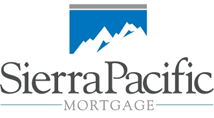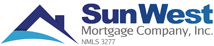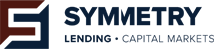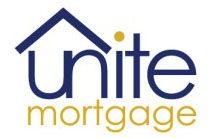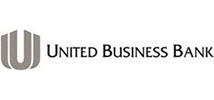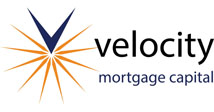## We Work With Over 80 Lenders

#### If you have any questions please contact us at 408-298-2591. You can also email us at <!-- function escramble(){ var a,b,c,d,e,f,g,h,i a='<a href=\"mai' b='<strong>info</strong>' c='\">' a+='lto:' b+='<strong>@</strong>' e='</a>' f='' b+='<strong>bayres.net</strong>' g='<img src=\"' h='' i='\" alt="Email us" border="0">' if (f) d=f else if (h) d=g+h+i else d=b document.write(a+b+c+d+e) } escramble() //--> .